Courses

# Test: nth Term of AP

## 20 Questions MCQ Test Mathematics (Maths) Class 10 | Test: nth Term of AP

Description
This mock test of Test: nth Term of AP for Class 10 helps you for every Class 10 entrance exam. This contains 20 Multiple Choice Questions for Class 10 Test: nth Term of AP (mcq) to study with solutions a complete question bank. The solved questions answers in this Test: nth Term of AP quiz give you a good mix of easy questions and tough questions. Class 10 students definitely take this Test: nth Term of AP exercise for a better result in the exam. You can find other Test: nth Term of AP extra questions, long questions & short questions for Class 10 on EduRev as well by searching above.
QUESTION: 1

### The common difference of an A.P. in which a25 – a12 = -52 is:

Solution:

We have an = a+(n-1)d
a25 = a+(25-1)d=a+24d
a12 = a+(12-1)d=a+11d
a25 - a12 = -52
a+24d-(a+11d)=-52
a+24d-a-11d=-52
13d= -52
d= -4

QUESTION: 2

### The number of two digit numbers divisible by 5 is

Solution:

Highest two digit number divisible by 5 is 95
l=95, a=10 and d=5
l=a+(n-1)d
95=10+(n-1)5
(n-1)5=85
n-1=17
n=18

QUESTION: 3

### Which term of the A.P. 1, 4, 7 … is 88?

Solution:
A+(n-1)d here a=1 and d=3 1+(n-1)3=88 1+3n-3)=88 -2+3n=88 3n=88+2 3n=90 so n=90/3 n=30.
QUESTION: 4

The 12th term of the A.P. with first term 1/2 and common difference 1/12 is …​

Solution:
QUESTION: 5

The common difference and the next two terms of the A.P are….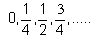Solution:

Common difference=¼-0=½-¼=¼
Next term =¾+¼=1
Next term =1+¼=5/4

QUESTION: 6

The next term of the given series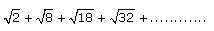would be

Solution:

We have an AP :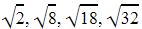Here a=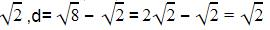l=a+(n-1)d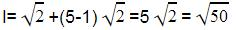QUESTION: 7

The common difference of the A.P for which 20th term is 10 more than the 18th term is :

Solution:
QUESTION: 8

The common difference of the AP for which 20th term is 10 more than the 18th term is:

Solution:
QUESTION: 9

The nth term of the AP 9, 13, 17, 21, 25, ………….. is:​

Solution:
QUESTION: 10

The common difference and the next two terms of the A.P are….1.8, 2.0, 2.2, 2.4,….​

Solution:
QUESTION: 11

The first negative term of the sequence 114, 109, 104 ………… is

Solution:

l=a+(n-1)d , d=109-114=-5
First negative term will be
0 > a+(n-1)d
0 > 114+(n-1)(-5)
0 > 114-5n+5
5n > 119
n > 119/5=23.8
So the first negative term will be 24th term.

QUESTION: 12

The common difference and the next two terms of the A.P are…. 75, 67, 59, 51,……​

Solution:
QUESTION: 13

If the sum of three consecutive terms of an increasing A.P. is 51 and the product of the first and third of these terms is 273, then the third term is

Solution: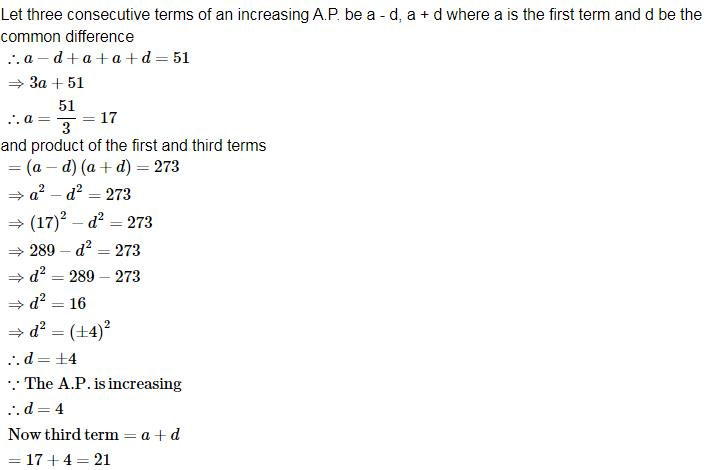QUESTION: 14

15th term of the A.P. x – 7, x – 2, x + 3 … is:​

Solution:

The given AP is x – 7, x – 2, x + 3 …
So a= x-7, d=x - 2- x + 7 = x + 3 - x +2 = 5
l = a + (n - 1)d
=x - 7 + (15 - 1)5
=x - 7 + 70 = x + 63

QUESTION: 15

Which term of A.P. 5, 13, 21, is 189

Solution:
QUESTION: 16

Which term of the A.P. 63, 84, …… is 399?​

Solution:

A=21

d=21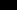=420

n=?=a+(n-1)d

420=21+(n-1)21

(n-1)*21=399

n-1=399/21

n-1=19

n=19+1=20

therefore the 20th term of the AP is 420

QUESTION: 17

In given AP 210 is …………. th term of: 2,6,10……..​

Solution:

We have a=2, d=6-2=4
l=a+(n-1)d
210=2+(n-1)4
208/4=n-1
n=53rd term

QUESTION: 18

How many terms are there in the AP 7, 13, 19, 25…205

Solution:

The sequence is an arithmetic sequence

The nth term of an AP is given by:

APn = a + d(n - 1)

a = first term = 7

d = common ratio = 13 - 7 = 6 or 19 - 13 = 6 or 25 - 19 = 6

205 = 7 + 6(n - 1)

6(n - 1) = 198

n - 1 = 33

n = 34

The sequence has 34 terms.

QUESTION: 19

The 11th term of 10.0, 10.5, 11.0, 11.5, ……is…​

Solution:

We have an AP :10.0, 10.5, 11.0, 11.5
Where a=10, d=0.5
l=a+(n-1)d
=10+(11-1)0.5
=10+5=15

QUESTION: 20

The 9th term of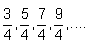is…​

Solution: